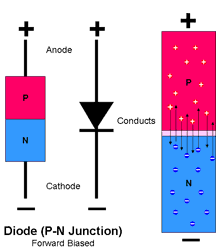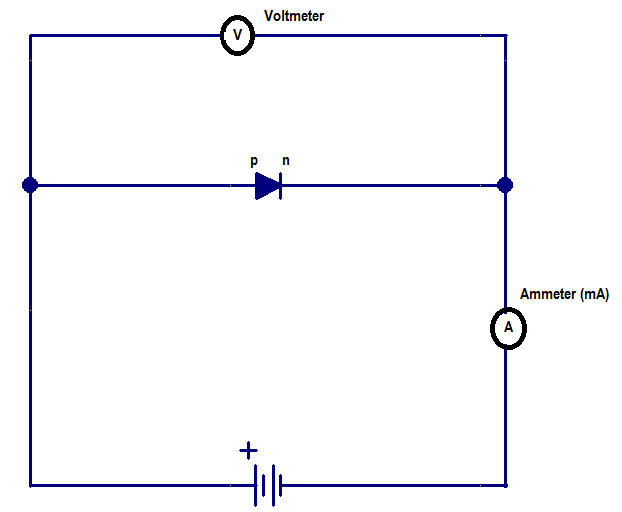Audio

[Audio][slideshow]

In chapter 1Understanding the PN junction , we have seen how a PN junction is formed from a p-type and n-type semiconductor. We have also learned about diffusion current, depletion region, drift current and barrier potential. If you find these terms foreign, just read the chapter about “understanding the pn junction” once more. Lets just make some questions. What is the use of a PN junction? Why have scientists created a pn junction device? What kind of problem it solves ? Learning anything is really fun when we question it. So these are our questions. Why there exists a pn junction in this world! ?;)

To get an answer to all these questions, lets first try to understand the characteristics of a PN junction. We know a pn junction has a “barrier potential”. Only if we overcome this “barrier potential” by applying an external voltage to the pn junction, we would be able to make it conducting. This simply means, current will pass through the pn junction only if we apply an external voltage higher than the “barrier potential” of pn junction. In chapter 1, we have seen that net current inside a pn junction is zero. Inorder to understand the behavior of a pn junction we need to make it conducting by applying an external voltage over a range (say from 0 volts 5 or 10 volts ), and then we study how the current passed through the pn junction varies with increasing voltage levels. To apply an external voltage, we usually connect 2 metallic contacts at the two ends of the pn junction (known as terminals); one on the p-side and other on the n-side. A PN junction with two metallic contacts is known as a pn junction diode or a semiconductor diode.

Note:- I have written an interesting article which tells the story behind invention & discovery of PN Junction diode. If you like to read the story, follow here:- Story behind Invention & Discovery of PN JunctionPN junction diode is symbolically represented as shown in picture. The direction of arrow is the direction of conventional current flow (under forward bias). Now lets try applying an external voltage to the pn junction diode. The process of applying an external voltage is called as “biasing”. There are two ways in which we can bias a pn junction diode.

1) Forward bias and 2) Reverse bias

The basic difference between a forward bias and reverse bias is in the direction of applying external voltage. The direction of external voltage applied in reverse bias is opposite to that of external voltage applied in forward bias.

Forward biasing a PN Junction diodeImage Source

Forward biasing a pn junction diode is very simple. You just need to take a battery whose values can be varied from (o to V volts), connect its positive terminal to the p-side of pn junction diode and then connect the negative terminal of battery to the n-side of the pn junction diode. If you have done upto this, the forward bias circuit of pn junction diode is complete. Now all we need to do is understand how the pn junction diode behaves when we increase the voltage levels from 0 to say 10 volts or 100 volts. We have learned that if we apply an external voltage higher than the barrier potential of pn junction diode, it will start conducting, which means it will start passing current through it. So how we are going to study the behavior of pn junction diode under forward biased condition? Lets get a voltmeter and ammeter and connect it to the forward biased circuit of pn junction diode.A simple circuit diagram is shown below, which has a pn junction diode, a battery (in picture it is not shown as variable. keep in mind we are talking about a variable power source), an ammeter (in milli ampere range) and a voltmeter.Note:- Assume that the pn junction diode is made from Silicon. The reason is difference in barrier potential for a diode made from Germanium and Silicon. (For a silicon diode – barrier potential is 0.7 volts where as for a Germanium diode barrier potential is low ~ 0.3 volts)

How to plot the characteristics of a pn junction ?

What we are going to do is, vary the voltage across diode by adjusting the battery. We start from o volts, then slowly move 0.1 volts, 0.2 volts and so on till 10 volts. Lets just note the readings of voltmeter and ammeter each time we adjust the battery (in steps of 0.1 volts). Finally after taking the readings, just plot a graph with voltmeter readings on X-axis and corresponding Ammeter readings on Y axis. Join all the dots in graph paper and you will see a graphical representation as shown below. Now this is what we call “characteristics of a pn junction diode” or the “behavior of diode under forward bias”Image Source

How to analyse the characteristics of a pn junction diode ?Image Source

Its from the “characteristics graph” we have just drawn, we are going to make conclusions about the behavior of pn junction diode. The first thing that we shall be interested in is about “barrier potential”. We talked a lot about barrier potential but did we ever mention its value ? From the graph, we observe that the diode does not conduct at all in the initial stages. From 0 volts to 0.7 volts, we are seeing the ammeter reading as zero! This means the diode has not started conducting current through it. From 0.7 volts and up, the diode start conducting and the current through diode increases linearly with increase in voltage of battery. From this data what you can infer ? The barrier potential of silicon diode is 0.7 volts 😉 What else ? The diode starts conducting at 0.7 volts and current through the diode increases linearly with increase in voltage. So that’s the forward bias characteristics of a pn junction diode. It conducts current linearly with increase in voltage applied across the 2 terminals (provided the applied voltage crosses barrier potential).

What happens inside the pn junction diode when we apply forward bias ?

We have seen the characteristics of pn junction diode through its graph. What really happens inside the diode during the forward bias ? We know a diode has a depletion region with a fixed barrier potential. This depletion region has a predefined width, say W. This width will vary for a Silicon diode and a Germanium diode. The width highly depends on the type of semiconductor used to make pn junction, the level of doping etc. When we apply voltage to the terminals of diode, the width of depletion region slowly starts decreasing. The reason for this is, in forward bias we apply voltage in a direction opposite to that of barrier potential. We know the p-side of diode is connected to positive terminal and n-side of diode is connected to negative terminal of battery. So the electrons in n-side gets pushed towards the junction (by force of repulsion) and the holes in p-side gets pushed towards the junction. As the applied voltage increases from 0 volts to 0.7 volts, the depletion region width reduces from ‘W’ to zero. This means depletion region vanishes at 0.7 volts of applied voltage. This results in increased diffusion of electrons from n-side to p-side region and the increased diffusion of holes from p-side to n-side region. In other words, “minority carrier” injection happens on both p-side (in a normal diode (without bias) electrons are a minority on p-side) and n-side (holes are a minority on n-side) of the diode.

How current flow takes place in a pn junction diode ?

This is another interesting factor, to explain. As the voltage level increases, the electrons from n-side gets pushed towards the p-side junction. Similarly holes from p-side gets pushed towards the n-side junction. Now there arises a concentration gradient between the number of electrons at the p-side junction region and the number of electrons at the region towards the p-side terminal. A similar concentration gradient develops between the number of holes at the n-side junction region and the number of holes at region near the n-side terminal. This results in movement of charge carriers (electrons and holes) from region of higher concentration to region of lower concentration. This movement of charge carriers inside pn junction gives rise to current through the circuit.

Reverse biasing a PN junction diode

Why should we reverse bias a pn diode ? The reason is, we want to learn its characteristics under different circumstances. By reverse biasing, we mean, applying an external voltage which is opposite in direction to forward bias. So here we connect positive terminal of battery to n-side of the diode and negative terminal of the battery to p-side of the diode. This completes the reverse bias circuit for pn junction diode. Now to study its characteristics (change in current with applied voltage), we need to repeat all those steps again. Connect voltmeter, ammeter, vary the battery voltage, note the readings etc etc. Finally we will get a graph as shown.Analysing the revere bias characteristics

Here the interesting thing to note is that, diode does not conduct with change in applied voltage. The current remains constant at a negligibly small value (in the range of micro amps) for a long range of change in applied voltage. When the voltage is raised above a particular point, say 80 volts, the current suddenly shoots (increases suddenly). This is called as “reverse current” and this particular value of applied voltage, where reverse current through diode increases suddenly is known as “break down voltage“.

What happens inside the diode ?

We connected p-side of diode to negative terminal of battery and n-side of diode to positive terminal of battery. So one thing is clear, we are applying external voltage in the same direction of barrier potential. If applied external voltage is V and barrier potential is Vx , then total voltage across the pn junction will be V+Vx. The electrons at n-side will get pulled from junction region to the terminal region of n-side and similarly the holes at p-side junction will get pulled towards the terminal region of p-side. This results in increasing the depletion region width from its initial length, say ‘W’ to some ‘W+x’. As width of depletion region increases, it results in increasing the electric field strength.

How reverse saturation current occurs and why it exists ?

The reverse saturation current is the negligibly small current (in the range of micro amperes) shown in graph, from 0 volts to break down voltage. It remains almost constant (negligible increase do exist) in the range of 0 volts to reverse breakdown voltage. How it occurs ? We know, as electrons and holes are pulled away from junction, they dont get diffused each other across the junction. So the net “diffusion current” is zero! What remains is the drift due to electric field. This reverse saturation current is the result of drifting of charge carriers from the junction region to terminal region. This drift is caused by the electric field generated by depletion region.

What happens at reverse breakdown ?

At breakdown voltage, the current through diode shoots rapidly. Even for a small change in applied voltage, there is a high increase in net current through the diode. For each pn junction diode, there will be a maximum net current that it can withstand. If the reverse current exceeds this maximum rating, the diode will get damaged.